• 基于灰色关联分析法的葫芦岛市大气环境质量评价，初玲玲，刘志斌，本文叙述了灰色关联分析法原理及其在大气环境质量评价中的应用。采用灰色关联分析法对葫芦岛各县（市）区大气环境质量进行评价
• 灰色关联分析法在宫山咀水库水质评价中的应用，初玲玲，刘志斌，本文叙述了灰色关联分析法原理及其在水环境质量评价中的应用，它是通过断面水质的多个水质浓度因子与水质标准的距离分析，说明
• 提出了用于确定相似材料合理配方的正交设计试验灰色关联分析法,给出了该方法的原理、模型和计算步骤。实际应用表明该法不仅正确可行而且具有其它多指标正交设计试验分析方法不具备的优越性。
• 灰色关联分析法简介 灰色关联分析是一种**系统分析** 灰色关联分析的基本思想是根据序列曲线几何形状的相似程度来判断其联系是否紧密。曲线越接近，相应序列之间的关联度就越大，反之就越小。 比较少用 ...


文章目录
灰色关联分析法简介步骤画统计图，进行简单分析确定分析数列对变量进行预处理计算子序列中各个指标与对应母指标的关联系数计算灰色关联度得到结论
讨论评价类问题完整分析步骤（该方法）对指标进行正向化预处理得到母序列计算灰色关联度计算各个指标的权重计算得分得分归一化

灰色关联分析法简介
灰色关联分析是一种系统分析灰色关联分析的基本思想是根据序列曲线几何形状的相似程度来判断其联系是否紧密。曲线越接近，相应序列之间的关联度就越大，反之就越小。  比较少用
步骤
例：下表某地区国内生产总值的统计数据（以百万元计），问该地区从2000年到2005年之间哪一种产业对GDP总量影响最大
年份国内生产总值第一产业第二产业第三产业200019883868397632001206140884680820022335422960953200327504821258101020043356511157712682005380656118931352
画统计图，进行简单分析四个季度都呈上升趋势第二产业增幅较为明显第二产业与第三产业的差距慢慢变小后又逐渐增大……
确定分析数列
母序列（又称参考序列，母指标）：能反应系统行为特征的数据序列（类似于因变量Y，此处记为

X

0

X_0

​）子序列（又称比较序列，子指标）：影响系统行为的因素组成的数据序列（类似于自变量X，此处记为

(

x

1

,

x

2

,

…

,

x

n

)

(x_1,x_2,…,x_n)

）在本例中国内生产总值就是母序列，第一、第二、第三产业就是子序列
对变量进行预处理
目的：去量纲、缩小变量范围从而简化计算做法：先求出每个指标的均值，再用该指标中的每个元素除以该均值计算子序列中各个指标与对应母指标的关联系数母序列：

X

0

=

(

X

0

(

1

)

,

X

0

(

2

)

,

…

X

0

(

n

)

)

)

T

X_0=(X_0(1),X_0(2),…X_0(n)))^T

​
子序列：

{

X

1

=

(

x

1

(

1

)

,

x

1

(

2

)

,

…

x

1

(

n

)

)

T

X

2

=

(

x

2

(

1

)

,

x

2

(

2

)

,

…

,

x

2

(

n

)

)

T

…

…

X

m

=

(

x

m

(

1

)

,

x

m

(

2

)

,

…

,

x

m

(

n

)

)

T

\begin{cases}{X_1=(x_1(1),x_1(2),…x_1(n))^T}\\{X_2=(x_2(1),x_2(2),…,x_2(n))^T}\\……\\X_m=(x_m(1),x_m(2),…,x_m(n))^T \end{cases}

计算：记

a

=

m

i

n

i

m

i

n

k

∣

X

0

(

k

)

−

x

i

(

k

)

∣

a=min_imin_k|X_0(k)-x_i(k)|

为两极最小差，记

b

=

m

a

x

i

m

a

x

k

∣

X

0

(

k

)

−

x

i

(

k

)

∣

b=max_imax_k|X_0(k)-x_i(k)|

为两极最大差
则上表中

a

=

0.0628

,

b

=

0.186163024

a=0.0628,b=0.186163024

定义

γ

(

x

0

(

k

)

,

x

i

(

k

)

)

=

a

+

ρ

b

∣

x

0

(

k

)

−

x

i

(

k

)

∣

+

ρ

b

,

ρ

\gamma(x_0(k),x_i(k))=\Large \frac {a+\rho b}{|x_0(k)-x_i(k)|+\rho b},\rho

​一般取0.5
结果：例如：

γ

(

x

0

(

1

)

,

x

1

(

1

)

)

=

0.0628

+

0.5

∗

0.18613024

0.10414232

+

0.5

∗

0.18613024

\gamma(x_0(1),x_1(1))=\Large \frac {0.0628+0.5*0.18613024}{0.10414232+0.5*0.18613024}

=

0.4751452

=0.4751452

计算灰色关联度
定义

γ

(

X

0

,

X

i

)

=

1

n

∑

k

=

1

n

γ

(

X

0

(

k

)

,

X

i

(

k

)

)

\gamma(X_0,X_i)=\frac 1n\sum_{k=1}^{n}\gamma(X_0(k),X_i(k))

为

X

0

X_0

和

X

i

X_i

的灰色关联度
即求平均值

γ

(

X

0

,

X

1

)

=

0.5084

,

γ

(

X

0

,

X

2

)

=

0.6242

,

γ

(

X

0

,

X

3

)

=

0.7573

\gamma(X_0,X_1)=0.5084,\gamma(X_0,X_2)=0.6242,\gamma(X_0,X_3)=0.7573

​得到结论
通过比较三个子序列和母序列的灰色关联度可以得到结论：
该地区在2000年到2005年间的国内生产总值受到第三产业影响最大。
讨论
1、什么时候用标准化回归，什么时候用灰色关联分析？
当样本个数n较大时，一般使用标准化回归；当样本个数n较少时，才使用灰色关联分析
2、如果母序列有多个指标，应该怎么分析
例如

Y

1

,

Y

2

Y_1,Y_2

都是母指标，那么我们先计算

Y

1

Y_1

​与子序列的灰色关联度，再计算

Y

2

Y_2

与子序列的灰色关联度
评价类问题完整分析步骤（该方法）
对指标进行正向化
预处理
如前面的步骤进行预处理（先求出每个指标的均值，再用该指标中的每个元素除以该均值）得到矩阵

Z

n

x

m

=

(

Z

i

j

)

n

x

m

Z_{nxm}=(Z_{ij})_{nxm}

得到母序列
（若无实质母序列）将预处理后的矩阵的每一行取出最大值构成母序列（虚构的）
计算灰色关联度
得到

γ

1

,

γ

2

,

…

，

γ

m

\gamma_1,\gamma_2,…，\gamma_m

计算各个指标的权重

ω

1

=

γ

1

(

γ

1

+

γ

2

+

…

+

γ

m

)

,

ω

2

=

γ

2

(

γ

1

+

γ

2

+

…

+

γ

m

)

,

…

,

ω

m

=

=

γ

m

(

γ

1

+

γ

2

+

…

+

γ

m

)

\omega_1=\frac {\gamma_1}{(\gamma_1+\gamma_2+…+\gamma_m)},\omega_2=\frac {\gamma_2}{(\gamma_1+\gamma_2+…+\gamma_m)},…,\omega_m==\frac {\gamma_m}{(\gamma_1+\gamma_2+…+\gamma_m)}

计算得分
第k个评价对象得分：

S

k

=

∑

i

=

1

m

Z

k

i

∗

γ

i

(

k

=

1

,

2

,

3

,

…

,

n

)

S_k=\sum_{i=1}^mZ_{ki}*\gamma_i (k=1,2,3,…,n)

​
得分归一化

S

1

′

=

S

1

S

1

+

S

2

+

…

+

S

n

,

S

2

′

=

S

2

S

1

+

S

2

+

…

+

S

n

,

…

S

n

′

=

S

n

S

1

+

S

2

+

…

+

S

n

S'_1=\frac {S_1}{S_1+S_2+…+S_n},S'_2=\frac {S_2}{S_1+S_2+…+S_n},…S'_n=\frac {S_n}{S_1+S_2+…+S_n}


展开全文数学建模
• 依据灰色关联分析原理，编制了计算机程序，并分析了某气田11口气井腐蚀的主要影响因素。结果表明，流速、c02分压、产出水中HCOf离子含量等因素对腐蚀有显著的影响，并探讨了这些因素对腐蚀的具体影响。腐蚀速度υc...
• function [y] = relevancy( refer,compare,p,rank) %refer参考数列(行向量)，compare比较数列 %p为分辨系数，默认为0.5 %rank为列向量，反映参考数列与比较数列同...%y返回一个反映关联度的列向量 [a,b]=size(compar...
function [y] = relevancy( refer,compare,p,rank)
%refer参考数列(行向量)，compare比较数列
%p为分辨系数，默认为0.5
%rank为列向量，反映参考数列与比较数列同增同减时是相关还是不相关
%rank为1表示同增同减时是相关（默认），rank为0表示同增同减时是不相关
%y返回一个反映关联度的列向量
[a,b]=size(compare);
if(nargin<3)
p=0.5;
end
if(nargin<4)
rank=ones(a,1);
end
%参考数列，比较数列初始化
refer=refer/refer(1);
for i=1:a
if(rank(i)==1)
compare(i,:)=compare(i,:)/compare(i,1);
else
compare(i,:)=compare(i,1)./compare(i,:);
end
end
%计算差值
for i=1:a
for j=1:b
diff(i,j)=compare(i,j)-refer(j);
end
end
%计算关联度
diff=abs(diff);
for i=1:a
sum=0;
for j=1:b
max1=max(max(diff'));
min1=min(min(diff'));
sum=sum+(min1+p*max1)/(diff(i,j)+p*max1);%关联度计算公式
end
y(i,1)=sum/b;%关联度取平均
end

不知道为啥今天放不了公式，就先这样了
灰色关联分析是指对一个系统发展变化态势的定量描述和比较的方法，其基本思想是通过确定参考数据列和若干个比较数据列的几何形状相似程度来判断其联系是否紧密，它反映了曲线间的关联程度。
通常可以运用此方法来分析各个因素对于结果的影响程度，也可以运用此方法解决随时间变化的综合评价类问题，其核心是按照一定规则确立随时间变化的母序列，把各个评估对象随时间的变化作为子序列，求各个子序列与母序列的相关程度，依照相关性大小得出结论。
灰色关联分析的步骤
灰色关联分析的具体计算步骤如下：
第一步：确定分析数列。
确定反映系统行为特征的参考数列和影响系统行为的比较数列。反映系统行为特征的数据序列，称为参考数列。影响系统行为的因素组成的数据序列，称比较数列。
第二步，变量的无量纲化
由于系统中各因素列中的数据可能因量纲不同，不便于比较或在比较时难以得到正确的结论。因此在进行灰色关联度分析时，一般都要进行数据的无量纲化处理。主要有初值化处理和均值化处理两种方法。
第三步，计算关联系数
第四步，计算关联度
因为关联系数是比较数列与参考数列在各个时刻（即曲线中的各点）的关联程度值，所以它的数不止一个，而信息过于分散不便于进行整体性比较。因此有必要将各个时刻（即曲线中的各点）的关联系数集中为一个值，即求其平均值，作为比较数列与参考数列间关联程度的数量表示，关联度公式如下：
第五步，关联度排序
数值越大说明关联性越强
展开全文MATLAB
• 灰色关联分析（Grey Relation Analysis，GRA），是一种多因素统计分析的方法。简单来讲，就是在一个灰色系统中，我们想要了解其中某个我们所关注的某个项目受其他的因素影响的相对强弱，再直白一点，就是说：我们...
释名
灰色关联度分析（Grey Relation Analysis，GRA），是一种多因素统计分析的方法。简单来讲，就是在一个灰色系统中，我们想要了解其中某个我们所关注的某个项目受其他的因素影响的相对强弱，再直白一点，就是说：我们假设以及知道某一个指标可能是与其他的某几个因素相关的，那么我们想知道这个指标与其他哪个因素相对来说更有关系，而哪个因素相对关系弱一点，依次类推，把这些因素排个序，得到一个分析结果，我们就可以知道我们关注的这个指标，与因素中的哪些更相关。
（ note ： 灰色系统这个概念的提出是相对于白色系统和黑色系统而言的。这个概念最初是由控制科学与工程（hhh熟悉的一级学科）的教授邓聚龙提出的。按照控制论的惯例，颜色一般代表的是对于一个系统我们已知的信息的多少，白色就代表信息充足，比如一个力学系统，元素之间的关系都是能够确定的，这就是一个白色系统；而黑色系统代表我们对于其中的结构并不清楚的系统，通常叫做黑箱或黑盒的就是这类系统。灰色介于两者之间，表示我们只对该系统有部分了解。）
举例
为了说明灰色关联度分析的应用场景，我们利用下图进行说明：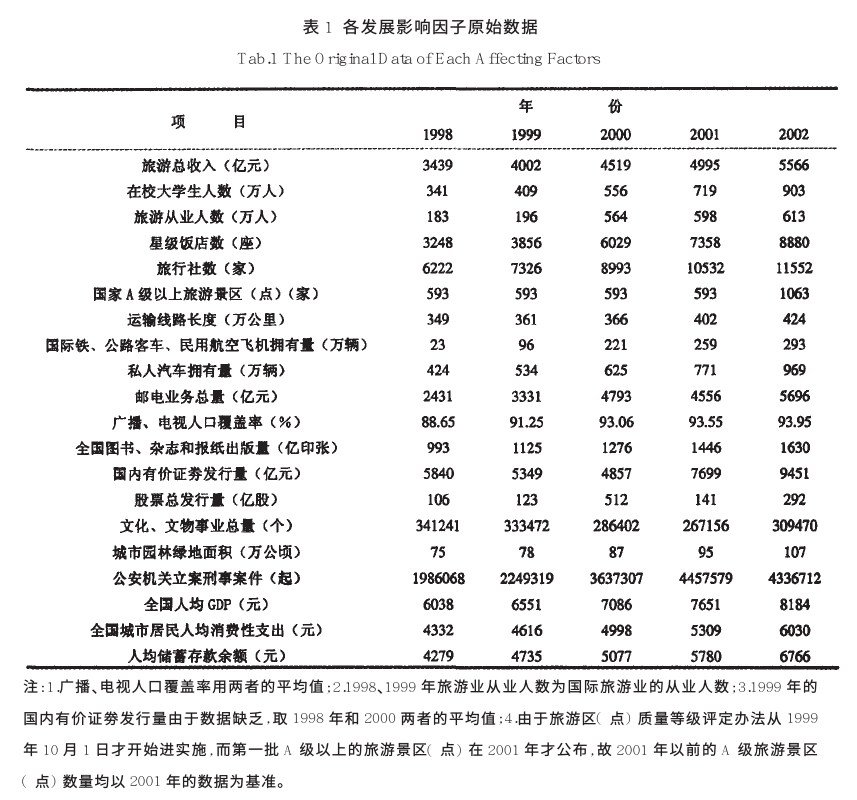该图来源于参考文献1。这篇文献研究的内容是旅游业发展的影响因子，看该表格，第一行为五年的旅游总收入，代表着旅游业发展的程度，而下面的这些要素就是我们需要分析的因子，比如在校大学生数，旅行社数，星级饭店数，A级景区数等等。最终目的是要得到一个排序，从而说明这些因子对旅游总收入的关联性的程度。
操作步骤与原理详解
（1） 确立母序列
（参考序列，在上面栗子中就是1998~2002年的旅游总收入序列）和子序列（比较序列，也就是需要确立顺序的因素序列，上栗中的除了第一行以外的所有因素都可以作为参考序列）
为了后面的表述方便，这里统一一下notation：
我们用x_i(k)表示第i个因素的第k个数值
用上面的栗子来说
比如第一个因素是在校大学生人数，那么x_1(1)就表示在校大学生人数在1998年的取值，也就是341，x_1(2)就是1999年的取值，
而x_2(1)就是表示旅游从业人数在1998年的数值。以此类推。
我们用x_0(k)表示母序列，i≥1的表示子序列，也就是要分析的要素的序列。
如果不写括号，比如x_i ，就代表这个元素的整个序列，也就是向量 x_i = [x_i(1), x_i(2), ... , x_i(n)]
n为每个向量的维度，也就是每个元素的特征的数量，在上栗中，n就是5，因为有五年的数据，代表五维向量。
以下所有表述都用该notation表示。

详解： 这个就是我们任务的目的（找到子序列和参考序列的关联程度），所以不需要再解释了吧～
（2）归一化，或者叫 无量纲化。
详解： 因为我们的这些要素是不同质的东西的指标，因此可能会有的数字很大有的数字很小，但是这并不是由于它们内禀的性质决定的，而只是由于量纲不同导致的，因此我们需要对它们进行无量纲化。这个操作一般在数据处理领域叫做归一化（normalization），也就是减少数据的绝对数值的差异，将它们统一到近似的范围内，然后重点关注其变化和趋势。 如下图所示，这是上面表格中前3个元素随年的变化曲线，以及作为母序列的旅游总收入：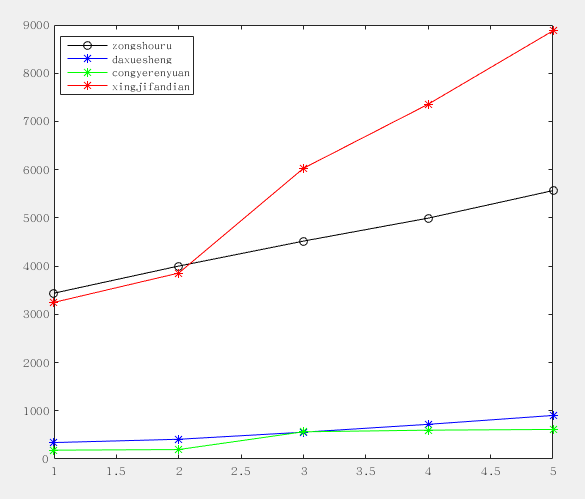可以看到，有两个曲线绝对数值很大，而另外两个很小，如果不做处理必然导致大的数值的影响会”淹没“掉小数值的变量的影响。
所以我们要对数据进行归一化处理，主要方法有如下几个：
（1） 初值化： 顾名思义，就是把这一个序列的数据统一除以最开始的值，由于同一个因素的序列的量级差别不大，所以通过除以初值就能将这些值都整理到1这个量级附近。
公式： x_i(k)' = x_i(k) / x_i(1)   i = 1,...,m, k = 1,...,n
(m为因素个数，n为每个因素的数据维度，仍如上栗，n=5，m=3（我们只看前三个因素，就是曲线图里画的这三种，和旅游总收入的关联，数据维度为5，即五年）)

（2） 均值化： 顾名思义，就是把这个序列的数据除以均值，由于数量级大的序列均值比较大，所以除掉以后就能归一化到1的量级附近。
公式： x_i(k)' = x_i(k) / ( mean(x_i) )        （除以均值）
其中 ： mean(x_i) = (1/n) sum_k=1^n (x_i(k))     （求第i个因素序列的均值）

其余还有如区间化，即把序列的值规范到一个区间，比如[0, 1]，之间。这个方法实际上在数据处理中应用比较多，但是在GRA中似乎常用均值化或者初值化，所以在此不介绍。
这里我们按照参考文献中的采用的方法，用初值化进行归一化，得到的结果如下图：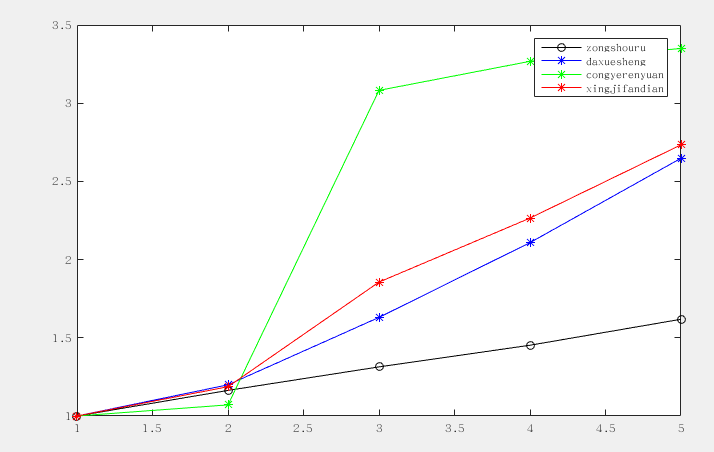可以看到，归一化以后的数据，量级差别变小了，这是为了后面提供铺垫，因为我们关注的实际上是曲线的形状的差异，而不希望绝对数值对后面的计算有影响。
（3）计算灰色关联系数
先放上公式：详解： 首先，我们把i看做固定值，也就是说对于某一个因素，其中的每个维度进行计算，得到一个新的序列，这个序列中的每个点就代表着该子序列与母序列对应维度上的关联性（数字越大，代表关联性越强）。 仔细观察这个公式，rho是一个可调节的系数，取值为(0,1)，大于零小于一，这一项的目的是为了调节输出结果的差距大小，我们放在后面讲。我们先假设把rho取成0，那么，这个式子就变成了
pseudo_zeta_i(k) = min min |x_0(k) - x_i(k)| / |x_0(k) - x_i(k)| = constant / |x_0(k) - x_i(k)|

我们看上面这个式子，可以发现，分子上这个数值，对于所有子序列来说都是一样的（，分子上这个数实际上就是所有因素的所有维度中，与母序列（参考序列，即我们要比较的序列）距离最近的维度上的距离。为什么要这样做呢？这样来想，假如我们没有进行归一化，或者不是用的初值化，而是用的均值化或者其他方法，可能会导致曲线之间，也就是母序列和各个子序列之间仍然有一段距离，那么这个距离最小值与下面的每个维度的距离相除，实际上也可以看成是一种取消量纲的手段。对于所有子序列，这个分子是相同的，所以实际上，这个系数pseudo_zeta是与第k个维度上，子序列与母序列的距离（差的绝对值，通常叫做l1范数（l1-norm））成反比，也就是说，这两个数距离越远，我们认为越不相关，这是符合直觉的。
当然，如果用了初值化归一化数据，如上面的图2所示，min min |x_0(k) - x_i(k)| 对每个i都会变成0，这样就不好了，因为这样一来，所有的zeta_i(k)都成了0，是无意义的。所以这时候我们就看到后面的 rho max max这一项的作用了。这一项对于每个i来说也是一个不变的常数constant，所以可以理解为给上面那个式子的分子分母同时加上某个数值，如下所示：
zeta_i(k) = (aconstant + bconstant) / (|x_0(k) - x_i(k)| + bconstant)

这样做的目的是什么呢？ 我们举个栗子： 对于两个分数： 1/5 和 1/4 ，它们的分子一样，分母相差为1，这时候他们的值相差1/20，也就是0.05，这就是没有＋rho max max那一项的情况，分子相同，分母的差代表着与参考序列的距离。 如果我们给他们分子分母同时加上20，那么就是21/25和21/24，它们相差为0.035，可以看到，加入这一项会导致同样的距离的点的系数差，会因为计算而变小。很显然地，rho取得越大，不同zeta系数的差距就越小。
另外，由于分子上是min min，也就是距离的全局最小值，这就导致下面的分母必然大于分子（不考虑 rho max max 项），而且，如果分母非常大，曲线距离非常远，那么，zeta接近0； 相反，如果x_i和x_0在所有维度上的差完全一样，那么分数的值就是1。这样zeta取值范围就是0~1之间，0表示不相关，1表示强关联性。这也符合认知。考虑上rho max max 项之后，我们知道对于一个真分数，分子分母都加一个同样的值，仍然是真分数（实际上是一个添加溶质的溶液的问题）。也就是说，仍然是0到1。
总结来说，rho是控制zeta系数区分度的一个系数，rho取值0到1， rho越小，区分度越大，一般取值0.5较为合适。zeta关联系数取值落在0到1之间。
接上栗，我们对上述三个子序列做出关联系数zeta的序列，结果如下：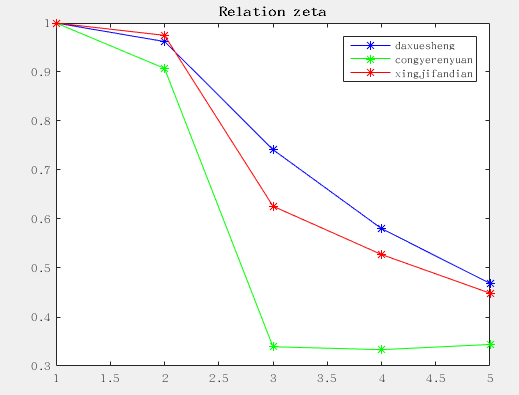其实从这个图中已经可以看出，大学生这一因素对旅游也的相关性普遍要高一些，从业人员相对影响少一些。星级饭店的数量居中。
(4) 计算关联系数均值，形成关联序
根据上图其实已经可以看出大概的趋势，但是这只是因为这个恰好所有维度上的趋势比较一致，实际上，我们得到zeta关联系数的值以后，应该对每个因素在不同维度上的值求取均值，换句话说，也就是对于上面那些zeta 的曲线，同一个颜色的求取均值。结果如下：
>> mean(zeta_1)
ans =
0.7505
>> mean(zeta_2)
ans =
0.5848
>> mean(zeta_3)
ans =
0.7154

可以看到，根据关联系数大小，排序结果为：
大学生人数 ＞ 星级饭店数量 ＞ 从业人员人数
这和参考文献论文中的结论是一致的：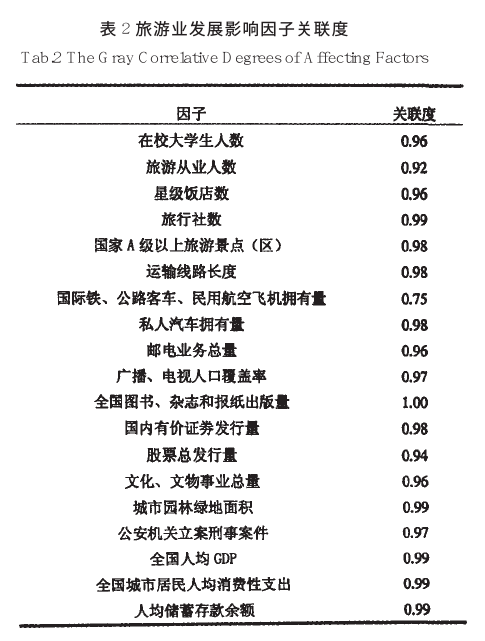（由于论文中用了所有的因子，导致max max 这个全局最大值不同，所以计算出的关联度数值与复现计算的结果数值不一样，但是这三个因子的关联度的排序是一致的，说明关联度是一个相对的指标，它反映的是不同因子与参考内容的关联程度）
总结
GRA算法本质上来讲就是提供了一种度量两个向量之间距离的方法，对于有时间性的因子，向量可以看成一条时间曲线，而GRA算法就是度量两条曲线的形态和走势是否相近。为了避免其他干扰，凸出形态特征的影响，GRA先做了归一化，将所有向量矫正到同一个尺度和位置，然后计算每个点的距离。最后，通过min min 和max max 的矫正，使得最终输出的结果落在0到1之间，从而符合系数的一般定义。rho调节不同关联系数之间的差异，换句话说，就是输出的分布，使其可以变得更加稀疏或者紧密。以数学角度要言之，该算法即度量已归一化的子向量与母向量的每一维度的l1-norm距离的倒数之和，并将其映射到0～1区间内，作为子母向量的关联性之度量的一种策略。
附录：MATLAB代码
下面是该博文中为举栗子复现的参考文献1中的计算过程MATLAB代码：
% Grey relation analysis

clear all
close all
clc

zongshouru = [3439, 4002, 4519, 4995, 5566];
daxuesheng = [341, 409, 556, 719, 903];
congyerenyuan = [183, 196, 564, 598, 613];
xingjifandian = [3248, 3856, 6029, 7358, 8880];

% define comparative and reference
x0 = zongshouru;
x1 = daxuesheng;
x2 = congyerenyuan;
x3 = xingjifandian;

% normalization
x0 = x0 ./ x0(1);
x1 = x1 ./ x1(1);
x2 = x2 ./ x2(1);
x3 = x3 ./ x3(1);

% global min and max
global_min = min(min(abs([x1; x2; x3] - repmat(x0, [3, 1]))));
global_max = max(max(abs([x1; x2; x3] - repmat(x0, [3, 1]))));

% set rho
rho = 0.5;

% calculate zeta relation coefficients
zeta_1 = (global_min + rho * global_max) ./ (abs(x0 - x1) + rho * global_max);
zeta_2 = (global_min + rho * global_max) ./ (abs(x0 - x2) + rho * global_max);
zeta_3 = (global_min + rho * global_max) ./ (abs(x0 - x3) + rho * global_max);

% show
figure;
plot(x0, 'ko-' )
hold on
plot(x1, 'b*-')
hold on
plot(x2, 'g*-')
hold on
plot(x3, 'r*-')
legend('zongshouru', 'daxuesheng', 'congyerenyuan', 'xingjifandian')

figure;
plot(zeta_1, 'b*-')
hold on
plot(zeta_2, 'g*-')
hold on
plot(zeta_3, 'r*-')
title('Relation zeta')
legend('daxuesheng', 'congyerenyuan', 'xingjifandian')

参考文献：
马晓龙. 旅游业发展影响因子灰色关联分析[J]. 人文地理, 2006, 21(2):37-40.谭学瑞, 邓聚龙. 灰色关联分析：多因素统计分析新方法[J]. 统计研究, 1995, 12(3):46-48.刘思峰, 蔡华, 杨英杰, et al. 灰色关联分析模型研究进展[J]. 系统工程理论与实践, 2013, 33(8):2041-2046.
2018年12月29日02:06:16 to Rita ～
展开全文• 基于灰色系统理论,研究了灰色关联分析法在系统综合评价中的应用。并通过实例对该方法进行了实证研究,表明了该方法的有效性
• 灰色关联分析(GRA)的理论及应用（matlab和python） 灰色关联分析模型(C++代码) 代码实现 maltab clc; close; clear all; x=xlsread('data1.xls'); %data1为网上参考数据格式，通过验证该程序没有问题，因此data也...
参考
灰色关联度分析（Grey Relation Analysis，GRA）原理详解灰色关联分析(GRA)的理论及应用（matlab和python）灰色关联分析模型(C++代码)
代码实现
maltab
clc;
close;
clear all;
x=x(:,2:end)'; %得到转置矩阵
column_num=size(x,2);   %列
index_num=size(x,1);     %行

% 1、数据均值化处理
x_mean=mean(x,2);  % 行向量均值
for i = 1:index_num
x(i,:) = x(i,:)/x_mean(i,1);
end
% 如果三个指标使用三种不同的归一化，则将上述四行代码改为以下形式：
%  x(1,:) = 第一个指标的归一化公式；
%  x(2,:) = 第二个指标的归一化公式；
%  x(3,:) = 第三个指标的归一化公式；

% 2、提取参考队列和比较队列
ck=x(1,:)   %第一行
cp=x(2:end,:)   %第二行以后所有
cp_index_num=size(cp,1);

%比较队列与参考队列相减
for j = 1:cp_index_num
t(j,:)=cp(j,:)-ck;
end
%求最大差和最小差
mmax=max(max(abs(t)))
mmin=min(min(abs(t)))
rho=0.5;

%3、求关联系数
ksi=((mmin+rho*mmax)./(abs(t)+rho*mmax))

%4、求关联度
weight = [1,0,0;0,1,0;0,0,1];
ksi_column_num=size(ksi,2);
r=sum(weight * ksi,2)/ksi_column_num;    %行求和

%5、关联度排序，得到结果r3>r2>r1
[GAG,rind]=sort(r,'descend');


python
import pandas as pd
x=x.iloc[:,1:].T

# 1、数据均值化处理
x_mean=x.mean(axis=1)
for i in range(x.index.size):
x.iloc[i,:] = x.iloc[i,:]/x_mean[i]

# 2、提取参考队列和比较队列
ck=x.iloc[0,:]
cp=x.iloc[1:,:]

# 比较队列与参考队列相减
t=pd.DataFrame()
for j in range(cp.index.size):
temp=pd.Series(cp.iloc[j,:]-ck)
t=t.append(temp,ignore_index=True)

#求最大差和最小差
mmax=t.abs().max().max()
mmin=t.abs().min().min()
rho=0.5
#3、求关联系数
ksi=((mmin+rho*mmax)/(abs(t)+rho*mmax))

#4、求关联度
r=ksi.sum(axis=1)/ksi.columns.size

#5、关联度排序，得到结果r3>r2>r1
result=r.sort_values(ascending=False)


c++
#include<iostream>
#include<cmath>
#define M 6	  //此处可进行行列数的修改
#define N 7
using namespace std;
int main()
{
//maxtrix of M N
double chushi[M][N];
double biaozhunhua[M][N];
cout << "input the matrix:\n";
for (int i = 0;i < M;i++)
for (int j = 0;j < N;j++)
cin >> chushi[i][j];
for (int i = 0;i < M;i++)
{
double Max = -1.0, Min = 10000;
for (int j = 0;j < N;j++)
{
if (chushi[i][j] > Max) Max = chushi[i][j];	//得到每行的最大值和最小值作为上下界
if (chushi[i][j] < Min) Min = chushi[i][j];
}
double jizhun = Max - Min;
for (int j = 0;j < N;j++)
biaozhunhua[i][j] = (chushi[i][j] - Min + 0.001) / (jizhun + 0.001);	//得到标准化矩阵,+0.01是为了防止除以0的情况
}
cout << endl;
//下面进行两级最小差与两级最大差的计算
//double liangji_max = -1, liangji_min = 10000;	//初始化
double temp_max = -1, temp_min = 10000;	//存放每行的一级最大值和一级最小值
for (int i = 0;i < M;i++)
{
//两两比较得出每行的一级最大值与一级最小值
double liangji_max = -1, liangji_min = 10000;	//初始化
for (int j = 0;j < N;j++)
{
for (int k = 0;k < N;k++) {
if (k == j) continue;
double t = fabs(biaozhunhua[i][j] - biaozhunhua[i][k]);
if (t > liangji_max) liangji_max = t;
if (t < liangji_min) liangji_min = t;
}
}
//赋值记录
temp_max = liangji_max;
if(liangji_min)
temp_min = liangji_min;
}
//cout << temp_max << ' ' << temp_min << endl;
//上面已经计算得出两级最大值和两级最小值
double rho = 0.5;
double fenzi = temp_min + rho * temp_max;
double guanlianxishu[M][N];
//下面依次计算每组数据的关联系数
for(int i = 0;i < M;i++)
{
for (int j = 0;j < N;j++)
{
double t = fabs(biaozhunhua[i][j] - 1.0);
double fenmu = t + rho * temp_max;
guanlianxishu[i][j] = fenzi / fenmu;
}
}
double weight[M] = { 0.29782,0.18947,0.24612,0.34625,0.1667,0.25383 };	//权重
double guanliandu[N];	//最终关联度
for (int i = 0;i < N;i++)	//对于每一列，计算其关联度
{
double sum = 0;
for (int j = 0;j < M;j++)
sum += weight[j] * guanlianxishu[j][i];
guanliandu[i] = sum;
}
cout << "1到7号飞机对应的最终关联度分别为：\n";
for (int i = 0;i < N;i++) cout << guanliandu[i] << ' ';
cout << endl;
system("pause");
return 0;
}


展开全文• ## 灰色关联度分析

千次阅读 多人点赞 2019-11-13 14:46:07
灰色关联分析 灰色关联分析方法步骤及原理； 另附例题及代码。Matlab
• 本笔记根据清风老师的课程以及《数学建模算法与应用》整理学习得到，如...灰色关联分析原理 灰色关联分析方法是基于灰色系统理论发展而来的一种综合评价和影响因素分析方法。 基本思想：通过对动态过程发展趋势...
• 运用灰色系统理论和矢量投影原理 ,提出了灰色关联投影 ,介绍了该方法的基本原理 ,给出了灰色关联投影角、灰色关联投影值等基本概念 ,总结了用该方法进行系统分析的一般...
• 首先对灰色关联分析原理和计算进行了介绍,然后以具体的露天矿山抛掷爆破工程的设计数据和实测数据为分析样本,通过MATLAB软件进行灰色关联矩阵计算,得出了影响爆破振动因素的主次序及关联度,并对露天矿山降震所采取...
• 灰色关联分析的基本思想是根据序列曲线几何形状的相似程度来判断其联系是否紧密。曲线接近，相应序列之间的关联度就越大，反之就越小 在建模中的应用场景 用于系统分析：例如分析粮食生产系统，人们总是希望提高...
• 本专栏第23篇数学建模学习笔记（二十三）灰色关联分析记录了灰色关联分析的一些基本知识。本篇内容对数学原理不作赘述，对matlab程序进行一定的补充。 灰色关联分析是国内学者提出的分析方法，适用于样本量比较少的...
• 灰色关联分析（Grey Relation Analysis，GRA），是一种多因素统计分析的方法，是一种对影响目标序列的相关因素进行定量分析的模型。 本文采用一个示例，简单分析一下。 数据集 链接: ...python
• 灰色关联分析对样本量要求小，样本量小的时候可以采用，但由于其是国人发明、且推广程度小，so 美赛不可以用，美赛只可以用数理统计分析。 画图简单分析 确定分析数列 ​2个序列正如其名，母是直观体现，...数学建模
• 1，灰色关联度分析（Grey Relation Analysis，GRA）原理详解 2，灰色关联分析法 3，灰色关联分析(GRA)的理论及应用（matlab和python） 4Python实现 灰色关联分析 与结果可视化 ...
• 分析了运输机的任务能力、短距起落能力、可用性、生存性、经济可承受性、研制风险 和舒适性等指标,运用灰色关联投影建立6种设计方案对评价指标集的加权灰色关联判断矩 阵,然后利用矢量投影原理计算了各设计方案...
• ## 数学建模学习笔记：灰色关联分析

千次阅读 多人点赞 2020-04-22 21:28:01
灰色关联分析概述 系统分析：一般的抽象系统包含很多影响因素，多种因素的共同作用结果决定了该系统的发展态势。如社会系统、经济系统、农业系统、生态系统、教育系统等等。 多因素之间的分析：哪些是主...
• 这一篇就简单介绍一下灰色关联分析吧。灰色关联分析主要有两个作用，一是进行系统分析，判断影响系统发展的因素的重要性。第二个作用就是用于综合评价问题，给出研究对象或者方案的优劣排名。 不过这里我只能简单...数学建模
• 灰色关联分析（Grey Relation Analysis，GRA），是一种多因素统计分析的方法。简单来讲，就是在一个灰色系统中，我们想要了解其中某个我们所关注的某个项目受其他的因素影响的相对强弱，再直白一点，就是说：我们...
• 采用灰色关联分析原理和方法,在以往研究影响条带煤柱及其覆岩破坏因素的基础上,利用灰色关联分析法构建了灰色关联度分析评价模型,以量化方法解决了评价指标因素排序问题,得出各影响因素的顺序,最终确定出主要影响...
• 本文旨在能快速地用Excel实现灰色关联分析，并简单地了解其原理。该系列文章是个人在参加2021年暑假国赛数模的培训，自己记录的心得与体会，意在总结归纳自己的学习成果，也希望能帮助想在数模比赛中施展身手的...数学建模
• 关联, 指的是关联分析, 这里引用百度百科的定义. 关联分析又称关联挖掘，就是在交易数据、关系数据或其他信息载体中，查找存在于项目集合或对象集合之间的频繁模式、关联、相关性或因果结构。 通过关联分析, 可以...
• 泥石流灾害的评估方法通常基于泥石流灾害预测理论的原理，已形成参数合成法、数理多元统计、层次分析法、信息模型法等多种评估预测模型。这些评估模型大多基于统计方法，其优点为指标体系的建立简单易行、可操作性较...
• 利用层次分析法,将成对比较矩阵的特征向量作为煤矿通风系统评价因子的权重,通过数据序列之间的灰色关联关系,建立灰色关联矩阵,以量化的方式准确地计算出各指标的影响力排序,最终指出影响矿井通风系统的主要因素。
• 在综合分析煤与瓦斯突出影响因素的基础上，利用灰色关联熵理论分析影响因素与突出危险性的关联度，得到各影响因素的权重及关联度排序，并结合概率神经网络（PNN）原理，构建基于灰色关联熵的煤与瓦斯突出PNN预测模型...概率神经网络
• 以北京市城区主要35种乔木树种为对象，从树木个体的景观美学角度去选择评价指标，用灰色关联度分析法对现有乔木树种进行...利用灰色关联分析方法评价城市景观树种，能客观、正确地反应各个树种的优劣，可操作性强，对于...